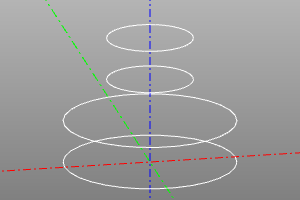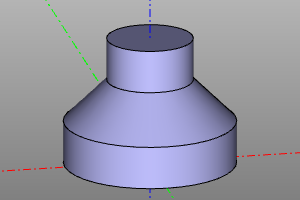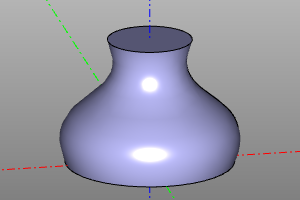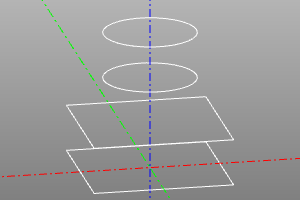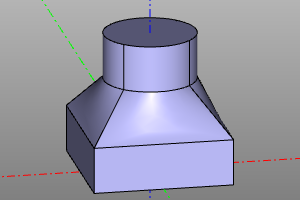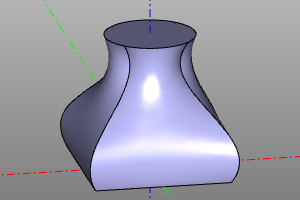# Reference geometry

## Offset

By displacing the shells, builds a thickened / thinned body based on the prototype proto and the radius of the thickening r. If r is positive, the shell is shifted outward, if negative - inward.

Signature:

``````offset(proto, r)
``````

Example:

``````offset(cone(r1=15,r2=10,h=20), r=5)
``````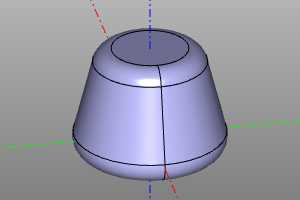## Ruled surface

Draws a face defined by a ruled surface based on the lines a, b.

Signature:

``````ruled(a, b)
``````

Example:

``````ruled(circle(r=20, wire=True), circle(r=20, wire=True).up(20))
ruled(circle(r=20, wire=True), circle(r=20, wire=True).rotZ(math.pi/2*3).up(20))
ruled(
interpolate([(0,0),(-4,10),(4,20),(-6,30),(6,40)]),
interpolate([(0,0),(-2,10),(2,20),(-4,30),(4,40)]).up(20),
)
``````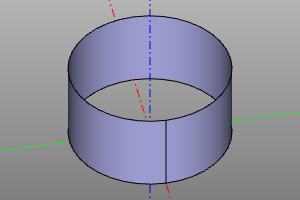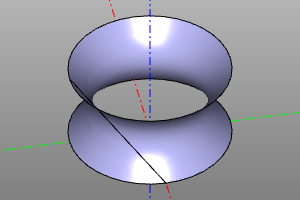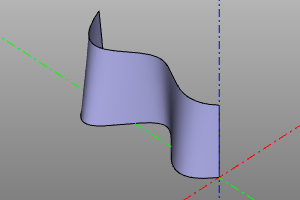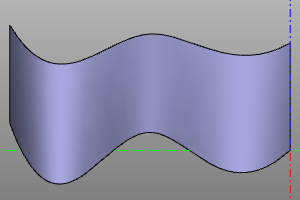## Binding

Constructing a shell on the profiles wireframe array. When the shell option is activated, a shell is built instead of a solid body. Activating the smooth option changes the ruled surface mode to a smooth approximation. When approximation is active, the maxdegree option limits the maximum degree of the polynomial.

Signature:

``````loft(profiles, smooth=False, shell=False, maxdegree=4)
``````

Example:

``````
``````